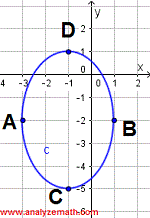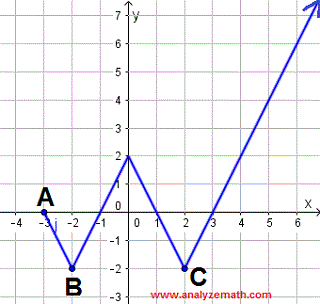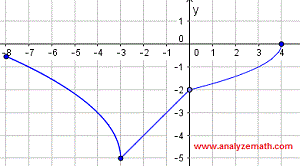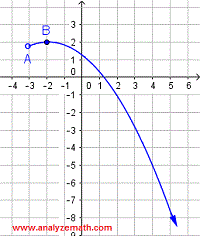# Find Domain and Range of Relations Given by Graphs Examples and Questions With Detailed Solutions

Find the domain and range of a relation given by its graph. Questions are presented along with detailed Solutions and explanations and also more questions with detailed solutions.

## Examples with Solutions

### Example 1

a) Find the domain and b) the range of the relation given by its graph shown below and c) state whether the relation is a function or not.Solution:
a) Domain: We first find the 2 points on the graph of the given relation with the smallest and the largest x-coordinate. In this example the 2 points are A(-2,-4) and B(4,-6) (see graph above). The domain is the set of all x values from the smallest x-coordinate (that of A) to the largest x-coordinate (that of B) and is written as:
-2 ≤ x ≤ 4
The double inequality above has the inequality symbol ≤ at both sides because the closed circles at points A and B indicate that the relation is defined at these values of x.
b) Range: We need to find the coordinates of the 2 points on the graph with the lowest and the largest values of the y coordinate. In this example, these points are B(4,-6) and C(2,2). The range is the set of all y values between the smallest and the largest y coordinates and given by the double inequality:
-6 ≤ y ≤ 2
The inequality symbol ≤ is used at both sides because the closed circles at points B and C indicates the relation is defined at these values.
c) The relation graphed above is a function because no vertical line can intersect the given graph at more than one point.

### Example 2

Find the a) domain and a) range of the relation given by its graph shown below and c) state whether the relation is a function or not.Solution:
a) Domain: In this example points A(-3,-5) and B(8,4) have the smallest and the largest x-coordinates respectively, hence the domain is given by:
-3 ≤ x ≤ 8
The use of the symbol ≤ at both sides is due to the fact that the relation is defined at points A and B (closed circles at both points).
b) Range: Points A and B have the smallest and the largest values of the y-coordinate respectively. The range is given by the inequality:
- 5≤ y ≤ 4
The use of the symbol ≤ at both sides is due to the fact that the relation is defined at points A and B.
c) No vertical line can cut the given graph at more than one point and therefore the relation graphed above is a function.

### Example 3

Find the domain and range of the relation given by its graph shown below and state whether the relation is a function or not.Solution:
a) Domain: Points A(-3,-2) and B(1,-2) have the smallest and the largest x-coordinates respectively, hence the domain:
-3 ≤ x ≤ 1
The use of the symbol ≤ at both sides is due to the fact that the relation is defined at points A and B (closed circles at both points).
b) Range: Points C(-1,-5) and D(-1,1) have the smallest and the largest y-coordinate respectively. The range is given by the double inequality:
- 5≤ y ≤ 1
The relation is defined at points C and D (closed circles), hence the use of the inequality symbol ≤.
c) There is at least one vertical line that cuts the given graph at two points (see graph below) and therefore the relation graphed above is NOT a function.### Example 4

Find the domain and range of the relation given by its graph shown below and state whether the relation is a function or not.Solution:
a) Domain: Points A(-3,0) has the smallest x-coordinate. The arrow at the top right of the graph indicates that the graph continues to the left as x increases. Hence there is no limit to the largest x-coordinate of points on the graph. The domain is given by all values greater than or equal to the smallest values x = -3 and is written as:
x ≥ -3
The use of the symbol ≥ at because the relation is defined at points A (closed circle at point A).
b) Range: Points B and C have equal and smallest y-coordinates equal to -2. The arrow at the top right of the graph indicates that the y coordinate increases as x increases. Hence there is no limit to the y-coordinate and therefore the range is given by all values greater than or equal to the smallest value y = -2 and is written as:
y ≥ -2
The use of the inequality symbol ≥ is due to the fact that the relation is defined at y = -2 (closed circle at B and C).
c) There is no vertical line that cuts the given graph at more than one point (see graph below) and therefore the relation graphed above is a function.

### Example 5

Find the domain and range of the relation given by its graph shown below and state whether the relation is a function or not.Solution:
a) Domain: Points A(-2,-3) has the smallest x-coordinate. The arrow at the top right of the graph indicates that the graph continues to the left as x increases. Hence there is no limit to the largest x-coordinate of points on the graph. The domain is given by all values greater than the smallest values x = - 2 and is written as:
x > -2
We use of the inequality symbol > (with no equal) because the relation is not defined at points A (open circle at point A).
b) Range: Points A(-2,-3) has the smallest y-coordinate equal to - 3. The arrow at the top right of the graph indicates that the y coordinate increases as x increases. Therefore there is no limit to the y-coordinate. Hence the range is given by all values greater than the smallest value y = - 3 and is written as:
y > - 3
The inequality symbol > is used because the relation is not defined at y = - 3 (open circle at point A).
c) The graph represents a function because there is no vertical line that cuts the given graph at more than one point.

## More Questions

For each relation below, find the domain and range and state whether the relation is a function.
a)b)c)d)e)## Solutions to the Above Questions

a)### Solution

a) Domain: Points A(-8 , - 0.5) and B(4,0) have the smallest and the largest x-coordinate respectively. The domain is the set of all x values between the smallest x-coordinate (that of A) to the largest x-coordinate (that of B) and is written as:
- 8 ≤ x ≤ 4
Since the relation is defined at both points (closed circle) the inequality symbol ≤ is used.
b) Range: Points C(-3,-5) and B(4,0) have the smallest and largest y-coordinates respectively. Hence, the range is the set of all y values between the smallest and the largest y coordinates and given by the double inequality:
- 5 ≤ y ≤ 0
The inequality symbol ≤ is used at both sides the relation is defined at these y values (closed circles).
c) The relation graphed above is a function because no vertical line can intersect the given graph at more than one point.

b)### Solution

a) Domain: Points A(-2 , 4) and B(4,6) have the smallest and the largest x-coordinate respectively. The domain is the set of all x values from the smallest x-coordinate (that of A) to the largest x-coordinate (that of B) and is written as:
- 2 ≤ x ≤ 4
Closed circles at both point A and B hence the use of the inequality symbol ≤.
b) Range: Points C(2,-2) and B(4,6) have the smallest and largest y-coordinates respectively. Hence, the range is the set of all y values between the smallest and the largest y coordinates and given by the double inequality:
- 2 ≤ y ≤ 6
The inequality symbol ≤ is used at both sides the relation is defined at these y values (closed circle).
c) The relation graphed above is a function because no vertical line can intersect the given graph at more than one point.

c)### Solution

a) Domain: Point A(4 , 2) has the largest x-coordinate. As x decreases (moving left), the arrow at the top left indicates that there is no limit to the smallest value of the x-coordinate of any point on the given graph. The domain is the set of all x values smaller than 4 and is written as:
x ≤ 4
The closed circle at point A means the relation is defined at x = 4, hence use of the inequality symbol ≤.
b) Range: Points B(2,-2) and C(-2,-2) have the smallest (and equal) y-coordinates. The arrow on the top left indicates that as x decreases (moving left), the y coordinate of points on the graph increases without limit. Hence, the range is the set of all y values greater than or equal to -2 and is given by the inequality:
y ≥ -2
The inequality symbol ≤ is used because the relation is defined at y = 4 (closed circle).
c) The relation graphed above is a function because no vertical line can intersect the given graph at more than one point.

d)### Solution

a) Domain: Points A(-5 , -1) and B(1, -1) have the smallest and the largest x-coordinate respectively. The domain is the set of all x values between - 5 and - 1 and is given by:
- 5 ≤ x ≤ 1
The closed circle at points A and B means the relation is defined at x = - 5 and x = 1, hence use of the inequality symbol ≤ at both sides.
b) Range: Points C(-2,-3) and D(-2,1) have the smallest and the largest y-coordinates respectively. Hence, the range is the set of all y values between -3 and 1 and is given by:
-3 ≤ y ≤ 1
The inequality symbol ≤ is used because the relation is defined at both points (closed circle).
c) The relation graphed above is NOT a function because at least one vertical line intersects the given graph at two points as shown below.e)### Solution

a) Domain: Point A(-3 , 1.8) has the smallest x-coordinate. As x increases (moving right), the arrow at the bottom right, indicates that there is no limit to the largest value of the x-coordinate of any point on the given graph. The domain is the set of all x values greater than -3 and is written as:
x > -3
The open circle at point A means the relation is not defined at x = -3, hence use of the inequality symbol >.
b) Range: Points B(-2,2) have the largest y-coordinates. The arrow on the bottom right indicates that as x increases (moving right), the y coordinate of points on the graph decreases without limit. Hence, the range is the set of all y values smaller than or equal to 2 and is given by the inequality:
y ≤ 2
The inequality symbol ≤ is used because the relation is defined at y = 2 (closed circle at B).
c) The relation graphed above is a function because no vertical line can intersect the given graph at more than one point.